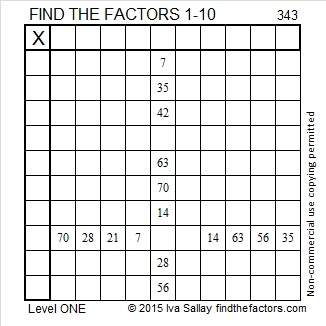# 343 and a Few Changes

Starting today, I’ve made a few small changes to the way my posts will look. If you can spot what the changes are, let me know if you have an opinion about them.

Quick Prime or Composite Number Test: If you divide 2^343 by 343, the remainder is 324, not 2, so 343 is NOT a prime number and is a composite number. (Use the mod function on the computer’s calculator to find the remainder.) Scroll down to see 343’s factors.Print the puzzles or type the factors on this excel file: 10 Factors 2015-01-05

• 343 is a composite number.
• Prime factorization: 343 = 7 x 7 x 7, which can be written 343 = 7³
• The exponent in the prime factorization is 3. Adding one, we get (3 + 1) = 4. Therefore 343 has exactly 4 factors.
• Factors of 343: 1, 7, 49, 343
• Factor pairs: 343 = 1 x 343 or 7 x 49
• Taking the factor pair with the largest square number factor, we get √343 = (√7)(√49) = 7√7 ≈ 18.520
• 343 is a perfect cube.(3+4)³ = 343, so 343 is a Friedman Number.